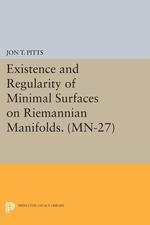# Existence and Regularity of Minimal Surfaces on Riemannian Manifolds. (MN-27):

Jon T. Pitts
Pages: 330
https://www.jstor.org/stable/j.ctt7zv66w

1. Front Matter
(pp. [i]-[iii])
(pp. [iv]-[vi])
3. INTRODUCTION
(pp. 1-11)

In this monograph, we develop a comprehensive variational calculus with which we explore the existence and regularity of minimal surfaces on riemannian manifolds. Our principal conclusion is the following theorem.

If 2 ≤ k ≤ 5, max{k,4} ≤ν≤ ∞, and M is a (k+1)-dimensional compact riemannian manifold of class (ν+1) , then M supports a nonempty, compact, k dimensional, imbedded, minimal submanifold (without boundary) of classν.

In these dimensions this theorem answers completely a more general question; namely, for what positive integers k and n does a smooth, compact, n dimensional, riemannian manifold support a regular...

4. CHAPTER 1 NONTECHNICAL SYSTEMIC DESCRIPTION AND ILLUSTRATIVE EXAMPLES
(pp. 12-47)

This chapter contains a reasonably complete but informal explanation of our methods, accomplished largely through examples and counterexamples. The reader may find this systemic summary useful in several ways. First, it provides an overview of the comprehensive theory which follows. The ideas which are the foundation of this monograph unify the structure and may be illustrated. Second, it motivates the theory. The finished product is written in considerable generality, and it is possible to lose sight of the simple natural origins of the concepts. For example, in chapters 3 and 4, we study a special generalized minimal surface (called an...

5. CHAPTER 2. PRELIMINARIES
(pp. 48-90)

Throughout this monograph we follow as closely as possible the standardized terminology of [FHl, pp. 669-671]. For varifolds, we have adopted most of the notation of [AW1]. Here we define our supplementary notation.

(1) Euclidean spaces and special subsets. We assume throughout that k, m, n, denote nonnegative integers. We denote by$\underline{\underline{\text{R}}},\ \overline{\underline{\underline{\text{R}}}},\ \underline{\underline{\text{Z}}},\ {{\underline{\underline{\text{Z}}}}_{\nu }},\ {{\underline{\underline{\text{R}}}}^{\text{n}}}$the real numbers, the extended real numbers$\underline{\underline{\text{R}}}\ \cup \,\{\infty ,-\infty \}$, the ring of integers, the ring of integers modulo$\nu \quad (\underline{\underline{\text{Z}}}/\nu \underline{\underline{\text{Z}}})$and the euclidean n dimensional space with its usual inner product · and norm | · |, respectively. Whenever$\text{p}\in {{\underline{\underline{\text{R}}}}^{\text{n}}}$and 0 ≤ s < r < ∞ , one...

6. CHAPTER 3. ALMOST MINIMIZING VARIFOLDS
(pp. 91-137)

The primary purpose of this monograph is to find surfaces which are regular solutions to certain naturally-posed variational problems. For emphasis one might say that there are two independent problems: first, to find solution surfaces (of any reasonable kind); and second, to find regular solution surfaces. In practice, however, these two problems are intertwined, and one prefers a unifying concept, provided of course that the concept is both natural and manageable. As we have undertaken this study, such a unifying concept has been a special kind of surface. We have called this surface an almost minimizing varifold, and it has...

7. CHAPTER 4. EXISTENCE OF ALMOST MINIMIZING VARIFOLDS
(pp. 138-177)

The principal theorem of this chapter is 4.11, establishing the existence of nonzero k dimensional almost minimizing varifolds on arbitrary smooth compact riemannian manifolds. We derive this existence theorem from a variational calculus based on generalized homotopy relations (4.1). These homotopy relations, motivated by the use of vietoris homology in [MM], are well suited for the "slice and replace with a comparison surface" arguments common in the theory of currents and varifolds, and are therefore easier to use here than conventional continuous homotopies.

The variational calculus was developed by Almgren in [AF2], where he used it to prove that riemannian...

8. CHAPTER 5. POINTWISE CURVATURE ESTIMATES FOR STABLE HYPERSURFACES
(pp. 178-223)

This chapter is primarily devoted to deriving pointwise curvature estimates for certain stable hypersurfaces: if$\text{M}\,\subset \,\text{N}\,\subset \,{{\underline{\underline{\text{R}}}}^{\text{n}}}$are properly imbedded submanifolds of class 4 of${{\underline{\underline{\text{R}}}}^{\text{n}}}$of dimensions k and k+1, respectively, if 2 ≤ k ≤ 5, if |M| is stable in N, and if M satisfies a modest mass hypothesis, then$\underline{\underline{\text{A}}}(\text{N,M)}$, the norm of the second fundamental form of M in N , is bounded pointwise by a number which depends on n and k, on bounds on the sectional curvatures of N and their derivatives, and on the mass of M. The complete theorem is stated...

9. CHAPTER 6. DECOMPOSITION THEOREM FOR STABLE HYPERSURFACES
(pp. 224-287)

Suppose k = 2,3,4, or 5, and$\text{M}\,\text{=}\,{{\underline{\underline{\text{R}}}}^{\text{k}+1}}$( or, more generally, M is a (k + 1) - dimensional submanifold of class 5 of${{\underline{\underline{\text{R}}}}^{\text{n}}}$for some n ≥ k + 1, and M is sufficiently planar). The decomposition theorem for stable hypersurfaces (6.3) is a precise description of the geometry of stable k dimensional submanifolds of M which lie near a cone. Complete specifications appear at 6.3, but the salient property is simply stated: in these dimensions a stable manifold sufficiently near a cone is the union of graphs of functions over a single k dimensional plane, the...

10. CHAPTER 7. REGULARITY
(pp. 288-326)

Let 2 ≤ k ≤ 5. This chapter contains the regularity theory for almost minimizing varifolds, the most important consequences of which are these.

(1) Every smooth compact riemannian manifold of dimension (k+1) supports a nonempty compact k dimensional minimal submanifold without boundary (7.13).

(2) We obtain in${{\underline{\underline{\text{R}}}}^{\text{k}+1}}$an interesting theorem of the type of Morse-Tompkins, Shiffman on the existence of (possibly unstable) regular k dimensional minimal surfaces spanning a(k-1)-dimensional cycle which bounds two distinct locally area minimizing currents (7.14).

(3) We prove an unusual compactness theorem (7.5): on a compact manifold, the space of mass-bounded stable k dimensional...

11. REFERENCES
(pp. 327-329)
12. Back Matter
(pp. 330-330)